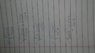# How to find the derivative of this function

rishi kesh

## Homework Statement

How do we find the derivative of function:
y= √[(1-sinx)/(1+sinx)]
This is the exercise problem from my textbook. I have not covered chain rule yet. So please you basic derivative rules to solve it.

## Homework Equations

Here is the answer of derivative given in my textbook: secx(tanx-secx)

## The Attempt at a Solution

I have tried replacing square root by power of 1/2 but can't reach to the answer.

Homework Helper
Gold Member
2022 Award

## Homework Statement

How do we find the derivative of function:
y= √[(1-sinx)/(1+sinx)]
This is the exercise problem from my textbook. I have not covered chain rule yet. So please you basic derivative rules to solve it.

## Homework Equations

Here is the answer of derivative given in my textbook: secx(tanx-secx)

## The Attempt at a Solution

I have tried replacing square root by power of 1/2 but can't reach to the answer.

Can you see a way to get rid of the square root?

•rishi kesh
rishi kesh
Can you see a way to get rid of the square root?

timetraveller123
think of manipulating the terms within the square root itself
(1 - sin^2x = cos ^2x)

•rishi kesh
Homework Helper
Gold Member
2022 Award
Whenever you have an expression of the form
##\frac{1+x}{1-x}##

you should think about multiplying top and bottom by ##1+x## or ##1-x##

•rishi kesh
rishi kesh
Whenever you have an expression of the form
##\frac{1+x}{1-x}##

you should think about multiplying top and bottom by ##1+x## or ##1-x##
Hi! I am sorry i tried but i couldn't solve it . I would greatly appreciate your help.

Homework Helper
Gold Member
2022 Award
Hi! I am sorry i tried but i couldn't solve it . I would greatly appreciate your help.
Multiply top and bottom by ##1-\sin x## and see what comes out.

rishi kesh
Multiply top and bottom by ##1-\sin x## and see what comes out.
Here is a picture of what I've solve. Please tell me if its correct.

#### Attachments

•1518543915303-2125212252.jpg
30.2 KB · Views: 472